# The Probability Line

Probability is all about chance. The probability that something will happen is the chance that it will happen. We can describe probability using words like

• Impossible
• Unlikely
• 50-50 chance
• Likely
• Certain
or using numbers. The probability of something impossible happening is $0$ and the probability of something certain happening is $1$. All other probabilities lie somewhere between $0$ and $1$. So, if someone tells you that the probability of something happening is bigger than $1$, like $1.5$ or $2$, then they're wrong.

We can represent probabilities on a line. It's a bit like a number line, but it only has values between $0$ and $1$. We'll first look at a line showing the words for probability and how they match up with the parts of the line from $0$ to $1$: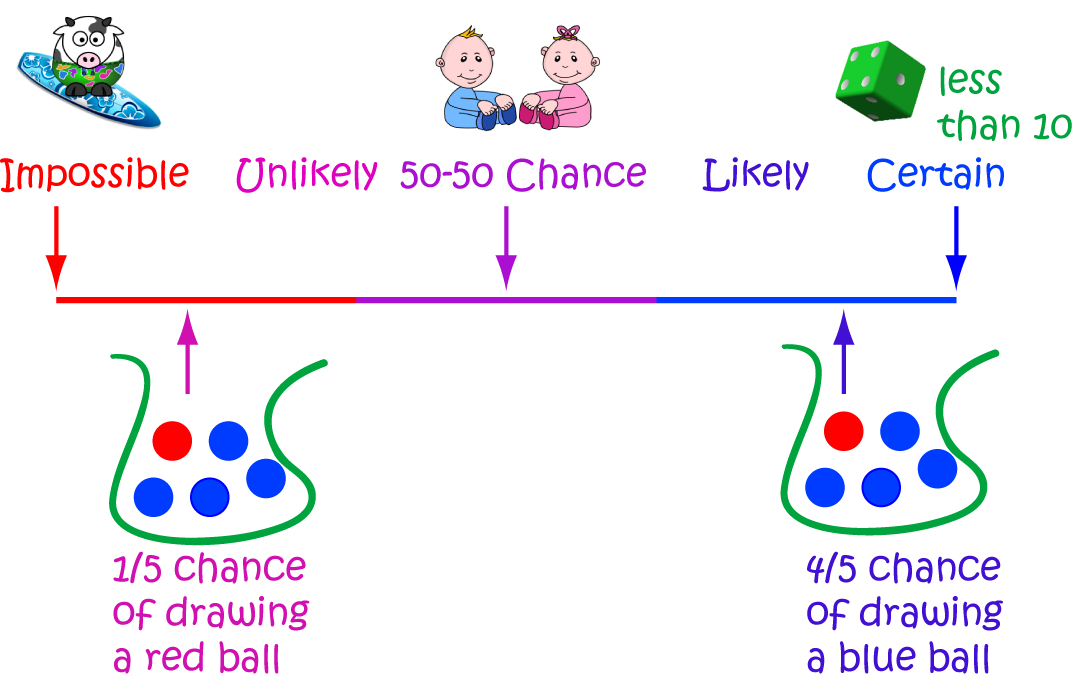So:

• It's impossible that you'll ever see a cow surfing.
• It is unlikely that you will draw a red ball out of a bag containing 1 red ball and 4 blue balls.
• If someone has a baby, there's a 50-50 chance that it will be a boy, and a 50-50 chance that it will be a girl.
• You are likely to draw a blue ball out of a bag containing 1 red ball and 4 blue balls.
• It is certain that if you roll a die (with the numbers 1 to 6 on it), then the number that lands face up will be less than 10.

We can also label our probability line with fractions like the one below: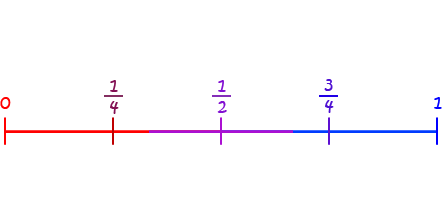Let's look at a few events and see if we place them along this line:

1. Tossing a coin so that it lands with either its head up or its tail up.
2. Choosing a yellow ball out of a bag containing only one blue ball and seven green balls: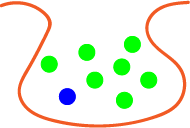3. Rolling a die and getting an even number ($2,4,6$)
4. Alice lands in the inflatable pool when she jumps off the very high platform shown below: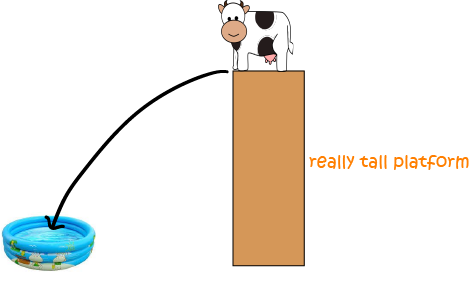5. The spinner shown below landing on yellowHow did you get on? Here's the line I got: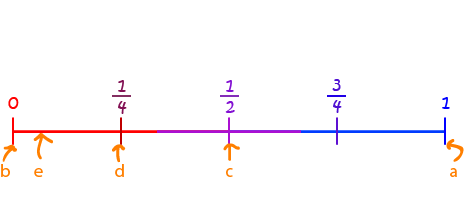And here's why:

1. Only two things can happen when you toss a coin: it either lands with heads or tails up. So, if I toss a coin, it's certain that it will land on either heads or tails. I've put this event down the certain end of the probability line.
2. The bag doesn't have any yellow balls in it, so it is impossible for me to draw a yellow ball out of it. This one goes right down at $0$.
3. Half the numbers on the die are even ($2,4,6$) and half of them are odd ($1,3,5$), so this event has a 50-50 chance of happening. I've put it in the middle of the probability line where you can see the fraction $\dfrac{1}{2}$.
4. I'm really worried about Alice and this circus trick. That little pool is so small and that platform is so high. I think it's highly unlikely that she'll land in the pool. In fact, it is close to impossible. I've put this one right down the end, almost at $0$, but not quite, because there is a small chance that she'll make it. I really hope so.
5. The spinner is divided evenly up into four colours, and yellow is one of them. So, there's a 1 in 4 chance that it will land on yellow. I've put this one at the fraction, $\dfrac{1}{4}$. It's definitely more likely that the spinner will land on yellow than that Alice will land in the pool.

## Summary

We can use a probability line to show how likely it is for different events to happen.

The probability line looks a bit like a number line, but it only shows numbers from $0$ to $1$ (or impossible to certain) because these are the only values the probability can take

• $0$ is the probability of an impossible event occurring.
• $1$ is the probability of a certain event occurring.

### Description

This mini book covers the core of Math for Foundation, Grade 1 and Grade 2 mathematics including

1. Numbers
3. Subtraction
4. Division
5. Algebra
6. Geometry
7. Data
8. Estimation
9. Probability/Chance
10. Measurement
11. Time
12. Money
13. and much more

This material is provided free of cost for Parent looking for some tricks for their Prekinder, Kinder, Prep, Year 1 and Year 2 children

### Learning Objectives

These lessons are for kids aged 4-8 with the core objective to expose their brains to concepts of addition, subtraction, division, algebra and much more.

Author: Subject Coach
You must be logged in as Student to ask a Question.